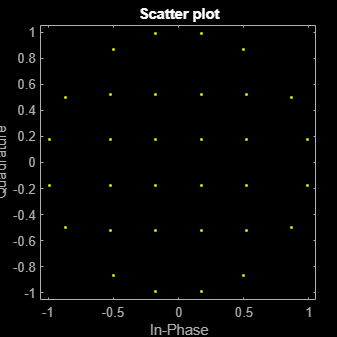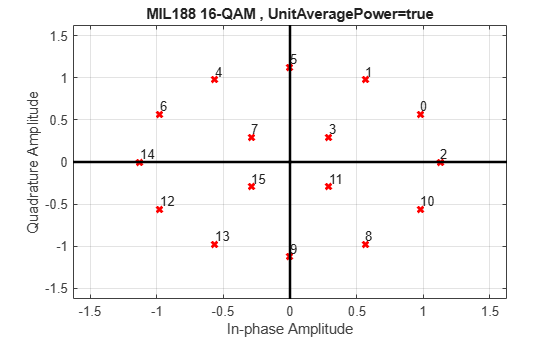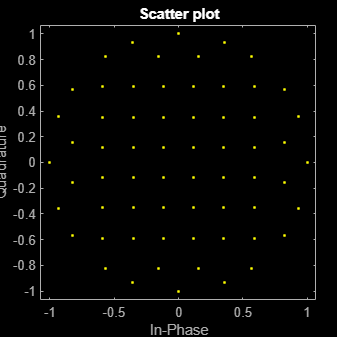# mil188qammod

MIL-STD-188-110 B/C standard-specific quadrature amplitude modulation (QAM)

## Syntax

``y = mil188qammod(x,M)``
``y = mil188qammod(x,M,Name,Value)``

## Description

example

````y = mil188qammod(x,M)` performs QAM modulation on the input signal, `x`, in accordance with MIL-STD-188-110 and the modulation order, `M`. For more information, see MIL-STD-188-110.```

example

````y = mil188qammod(x,M,Name,Value)` specifies options using one or more name-value pair arguments. For example, `'OutputDataType','double'` specifies the desired output data type as double. Specify name-value pair arguments after all other input arguments.```

## Examples

collapse all

Modulate data using 32-QAM as specified in the MIL-188-110C standard. Display the result using a scatter plot.

Set M to 32 and create a data vector containing all possible symbols.

```M = 32; x = (0:M-1);```

Modulate the data using QAM as specified in MIL-STD-188-110C.

`y = mil188qammod(x,M);`

Display the constellation as a scatter plot.

`scatterplot(y)`Modulate data using 16-QAM as specified in the MIL-STD-188-110B standard. Normalize the modulator output so that it has an average signal power of 1 W.

Set M and generate random data.

```M = 16; x = randi([0 M-1],1e5,1);```

Modulate the data applying 16-QAM as specified in MIL-STD-188-110B. Using name-value pairs, set the unit average power to `true` and enable the constellation plot.

`y = mil188qammod(x,M,'UnitAveragePower',true,'PlotConstellation',true);`Verify that the signal has approximately unit average power.

`avgPow = mean(abs(y).^2)`
```avgPow = 1.0012 ```

Modulate a sequence of bits using 64-QAM as specified by MIL-STD188-110B. Display the constellation.

Set the modulation order and generate a sequence of random bits.

```M = 64; numBitsPerSym = log2(M); data = randi([0 1],1000*numBitsPerSym,1);```

Modulate the data applying 64-QAM as specified by MIL-STD-188-110B, and output constellation symbols of single data type.

`y = mil188qammod(data,M,'InputType','bit','OutputDataType','single');`

Plot the result constellation using a scatter plot.

`scatterplot(y)`## Input Arguments

collapse all

Input signal, specified as a scalar, vector, or matrix. The elements of x must be binary values or integers that range from 0 to (`M` – 1), where `M` is the modulation order.

Note

To process input signal as binary elements, set the `'InputType'` value to `'bit'`. For binary inputs, the number of rows must be an integer multiple of log2(`M`). Groups of log2(`M`) bits in a column are mapped onto a symbol, with the first bit representing the MSB and the last bit representing the LSB.

Data Types: `double` | `single` | `int8` | `int16` | `int32` | `uint8` | `uint16` | `uint32` | `logical`

Modulation order, specified as a power of two. The modulation order specifies the total number of points in the signal constellation.

Example: `16`

Data Types: `double`

### Name-Value Arguments

Specify optional pairs of arguments as `Name1=Value1,...,NameN=ValueN`, where `Name` is the argument name and `Value` is the corresponding value. Name-value arguments must appear after other arguments, but the order of the pairs does not matter.

Before R2021a, use commas to separate each name and value, and enclose `Name` in quotes.

Example: y = mil188qammod(data,M,'InputType','bit','OutputDataType','single');

Input type, specified as the comma-separated pair consisting of `'InputType'` and either `'integer'` or `'bit'`. If you specify `'integer'`, the input signal must consist of integers from 0 to `M` – 1. If you specify `'bit'`, the input signal must contain binary values, and the number of rows must be an integer multiple of log2(`M`).

Data Types: `char` | `string`

Output data type, specified as the comma-separated pair consisting of `OutputDataType` and `'double'` or `'single'`.

Data Types: `char` | `string`

Unit average power flag, specified as the comma-separated pair consisting of `'UnitAveragePower'` and a logical scalar. When this flag is `true`, the function scales the constellation to an average power of 1 watt referenced to 1 ohm. When this flag is `false`, the function scales the constellation based on specifications in the relevant standard, as described in .

Data Types: `logical`

Option to plot constellation, specified as the comma-separated pair consisting of `'PlotConstellation'` and a logical scalar. To plot the constellation, set `PlotConstellation` to `true`.

Data Types: `logical`

## Output Arguments

collapse all

Modulated signal, returned as a complex scalar, vector, or matrix. The dimension of the output depends on the specified `InputType` value.

`InputType`Dimensions of Output
`'integer'``y` has the same dimensions as input `x`.
`'bit'`The number of rows in `y` equals the number of rows in `x` divided by log2(`M`).

Data Types: `double` | `single`